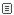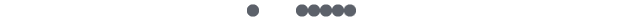##7个提升Python程序性能的好习惯

1、使用局部变量

2、减少函数调用次数

#判断变量num是否为整数类型

`1.     type(num) == type(0)   `
`2.     #调用三次函数  `
`3.     type(num) is type(0)   `
`4.     #身份比较  `
`5.     isinstance(num,(int))   `
`6.     #调用一次函数 `

`1.     #每次循环都需要重新执行len(a)  `
`2.     while i < len(a):  `
`3.         statement  `
`4.     #len(a)仅执行一次  `
`5.     m = len(a)  `
`6.     while i < m:  `
`7.         statement `

3、采用映射替代条件查找

`1.     #if查找  `
`2.     if a == 1:  `
`3.         b = 10  `
`4.     elif a == 2:  `
`5.         b = 20  `
`6.     ...  `
`7.     #dict查找，性能更优  `
`8.     d = {1:10,2:20,...}  `
`9.     b = d[a] `

4、直接迭代序列元素

`1.     a = [1,2,3]  `
`2.     #迭代元素 `
`3.     for item in a:     `
`4.     print(item)  `
`5.     #迭代索引  `
`6.     for i in range(len(a)):   `
`7.     print(a[i]) `

5、采用生成器表达式替代列表解析

`1.     #计算文件f的非空字符个数  `
`2.     #生成器表达式  `
`3.     l = sum([len(word) for line in f for word in line.split()])  `
`4.     #列表解析  `
`5.     l = sum(len(word)   `
`6.     for line in f for word in line.split()) `

6、先编译后调用

7、模块编程习惯评论发表评论